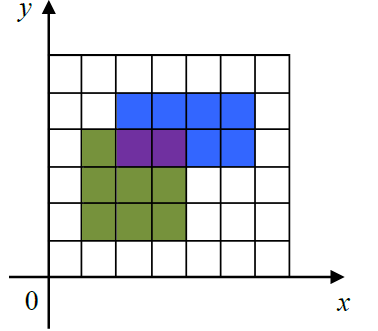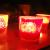## 一个画图题目，Java

newfresh 发布于 2014/12/08 15:16

在一个定义了直角坐标系的纸上，画一个(x1,y1)到(x2,y2)的矩形指将横坐标范围从x1到x2，纵坐标范围从y1到y2之间的区域涂上颜色。
下图给出了一个画了两个矩形的例子。第一个矩形是(1,1) 到(4, 4)，用绿色和紫色表示。第二个矩形是(2, 3)到(6, 5)，用蓝色和紫色表示。图中，一共有15个单位的面积被涂上颜色，其中紫色部分被涂了两次，但在计算面积时只计算一次。在实际的涂色过程中，所有的矩形都涂成统一的颜色，图中显示不同颜色仅为说明方便。输入的第一行包含一个整数n，表示要画的矩形的个数。
接下来n行，每行4个非负整数，分别表示要画的矩形的左下角的横坐标与纵坐标，以及右上角的横坐标与纵坐标。

输出一个整数，表示有多少个单位的面积被涂上颜色。

2
1 1 4 4
2 3 6 5

15

0```import java.util.Scanner;
import java.util.HashSet;
// import java.util.AbstractCollection;

/**
* 感觉我的算法投机取巧
* 把每个矩形分解成许多单位矩形，存入set
* 最后set中元素的个数即为总面积
*/
public class RecArea{
public static void main(String[] args){
Scanner sc = new Scanner(System.in);
int n = sc.nextInt(); //要画矩形的个数
HashSet<UnitGrid> set = new HashSet<UnitGrid>();
int[] axis = new int;
for(int i = 1; i <= n; i++){
for(int j = 0; j < 4; j++){
axis[j] = sc.nextInt();
}
set.addAll(transferToUniteGrid(axis, axis, axis, axis));
}
System.out.println(set.size());
}

private static HashSet<UnitGrid> transferToUniteGrid(int x1, int y1, int x2, int y2){
HashSet<UnitGrid> set = new HashSet<UnitGrid>();
//对两个点进行排序，以保证x1<x2,y1<y2。
int temp;
if(x1 > x2){
temp = x1;
x1 = x2;
x2 = temp;
}
if(y1 > y2){
temp = y1;
y1 = y2;
y2 = temp;
}
for(int i = x1; i < x2; i++){//不包括上界
for(int j = y1; j < y2; j++){
set.add(new UnitGrid(i,j));
}
}
return set;
}

}

class UnitGrid{
int x, y; //用左下角的坐标来代表一个UnitGrid
public UnitGrid(int x, int y){
this.x = x;
this.y = y;
}

//重写equals方法，若左下角坐标一致，则相等
@Override
public boolean equals(Object o){
if(o == null) return false;
if(!(o instanceof UnitGrid)) return false;
UnitGrid ug = (UnitGrid)o;
if((this.x == ug.x) && (this.y == ug.y)){
return true;
}
return false;
}

//重写hashCode方法。
@Override
public int hashCode(){
//如果两个UnitGrid的x,y相等，则为同一元素
int result = 17;
return (37*result + this.x)*37+this.y;
}
}```